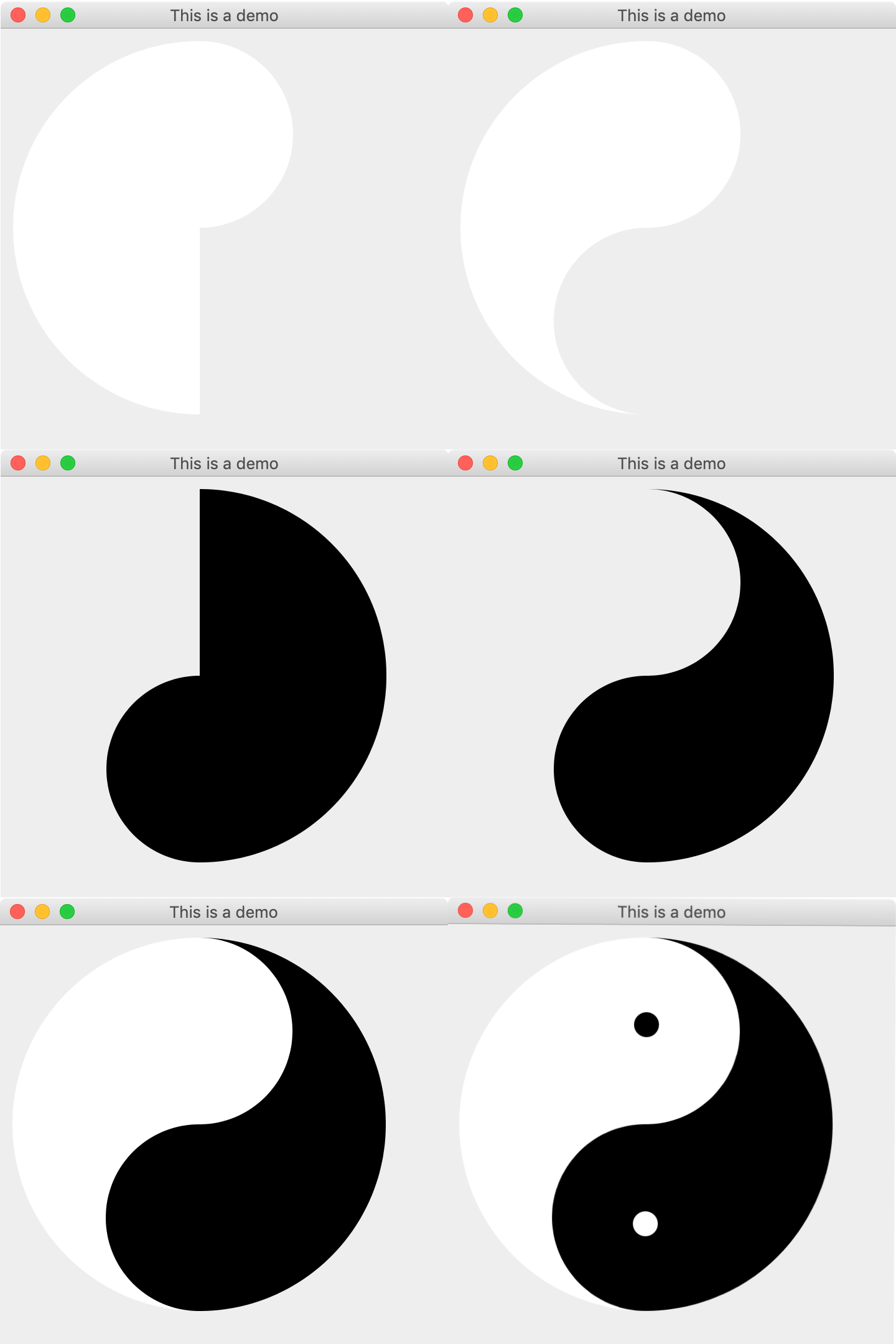1. 先画一个整圆，借助一个矩形，用`Subtraction`操作，获得一个半圆。
2. 再画一个小圆，用`Union`操作，得到下图左一图形。
3. 再画一个小圆，用`Subtraction`操作，得到下图右一图形。
4. 同理，得到下图右二图形。
5. 右一和右二，用`Union`操作，得到左三图形。
6. 最后再画上两个小圆即可。`123456789101112131415161718192021222324252627282930313233343536373839404142434445464748495051525354555657585960616263646566676869707172737475767778798081828384858687888990919293949596979899100101102103104` ``````import javax.swing.*; import java.awt.*; import java.awt.geom.Area; import java.awt.geom.Ellipse2D; import java.awt.geom.Rectangle2D; public class Java2DTest { public static void main(String[] args) { JFrame ui = new JFrame("TaiChi Graphics Demo"); ui.setDefaultCloseOperation(JFrame.EXIT_ON_CLOSE); ui.setPreferredSize(new Dimension(360, 360)); ui.getContentPane().setLayout(new BorderLayout()); ui.getContentPane().add(new CustomJPanel(), BorderLayout.CENTER); ui.getContentPane().add(new TaiChiDemo(), BorderLayout.CENTER); ui.pack(); ui.setVisible(true); } private static class TaiChiDemo extends JPanel { @Override protected void paintComponent(Graphics g) { Graphics2D g2d = (Graphics2D) g; g2d.setRenderingHint(RenderingHints.KEY_ANTIALIASING, RenderingHints.VALUE_ANTIALIAS_ON); // 绘制左侧图形 Area left = getLeftArea(); g2d.setPaint(Color.WHITE); g2d.fill(left); // 绘制右侧图形 Area right = getRightArea(); g2d.setPaint(Color.BLACK); g2d.fill(right); // 绘制中间两个小圆 Shape blackCircle = new Ellipse2D.Double(150,70, 20,20); g2d.setPaint(Color.BLACK); g2d.fill(blackCircle); Shape whiteCircle = new Ellipse2D.Double(150, 230, 20, 20); g2d.setPaint(Color.WHITE); g2d.fill(whiteCircle); g2d.dispose(); } public Area getLeftArea() { // 获取左半边的圆形 x,y 为左上角开始绘制的坐标点, w 为椭圆的宽度, h 为椭圆的高度 Shape leftHalfCircle = new Ellipse2D.Double(10, 10, 300, 300); // 定义左侧区域 Area left = new Area(leftHalfCircle); // 需要借助一个矩形来完成图形的减操作 Shape rightRectangle = new Rectangle2D.Double(160, 10, 150, 300); // 定义右侧矩形区域 Area rightRectangleArea = new Area(rightRectangle); // 获取上面内部圆形 Shape topInnerCircle = new Ellipse2D.Double(85, 10, 150, 150); Area topInnerCircleArea = new Area(topInnerCircle); // 获取下面内部圆形 Shape bottomInnerCircle = new Ellipse2D.Double(85, 160, 150, 150); Area bottomInnerCircleArea = new Area(bottomInnerCircle); // 对左侧图形进行操作 left.subtract(rightRectangleArea); left.add(topInnerCircleArea); left.subtract(bottomInnerCircleArea); return left; } public Area getRightArea() { // 获取右半边的圆形 x,y 为左上角开始绘制的坐标点, w 为椭圆的宽度, h 为椭圆的高度 Shape rightHalfCircle = new Ellipse2D.Double(10, 10, 300, 300); // 定义右侧区域 Area right = new Area(rightHalfCircle); // 需要借助一个矩形来完成图形的减操作 Shape leftRectangle = new Rectangle2D.Double(10, 10, 150, 300); // 定义左侧矩形区域 Area leftRectangleArea = new Area(leftRectangle); // 获取上面内部圆形 Shape topInnerCircle = new Ellipse2D.Double(85, 10, 150, 150); Area topInnerCircleArea = new Area(topInnerCircle); // 获取下面内部圆形 Shape bottomInnerCircle = new Ellipse2D.Double(85, 160, 150, 150); Area bottomInnerCircleArea = new Area(bottomInnerCircle); // 对右侧图形进行操作 right.subtract(leftRectangleArea); right.add(bottomInnerCircleArea); right.subtract(topInnerCircleArea); return right; } } } ``````